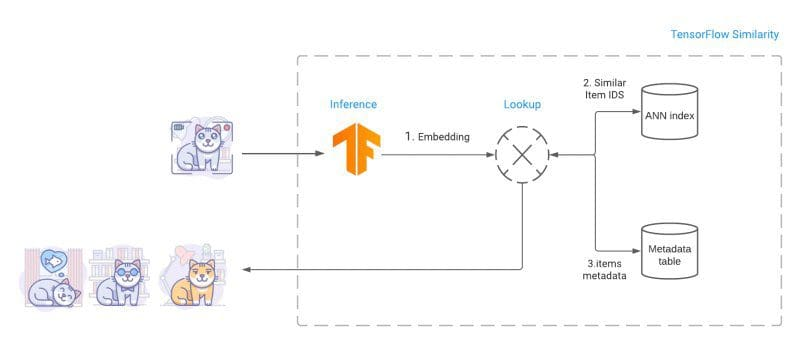# Introducing TensorFlow Similarity

TensorFlow Similarity is a newly-released library from Google that facilitates the training, indexing and querying of similarity models. Check out more here.

Often we need to be able to find things that are like other things. Similarity searching is a useful technique for doing so. In data science, contrastive learning can be used to build similarity models which can then be used for similarity searching.

Similarity models are trained to output embeddings in which items are embedded in a metric space, resulting in a situation where similar items are close to one another and further from dissimilar items. This is directly related — both intuitively and mathematically — to word embeddings, with which you are already familiar; Paris and London are close to one another, as are mustard and ketchup, but these 2 groups are comparatively further apart from one another.

In this same way, we could use a similarity model for comparing, say, vehicles. For example, BMW and Mercedes sedans would be embedded close to one another, while Yamaha and Kawasaki motorcycles would find themselves closely embedded. The motorcycles would be relatively further from the sedans, which would, in turn, be distanced from other dissimilar vehicles such as boats, motor homes, vans, bicycles, etc.Similarity model overview (source)

Deep learning is used to train the neural networks which often find themselves being used for these similarity models. To facilitate the training of such models, Google has very recently released TensorFlow Similarity.

Tensorflow Similarity offers state-of-the-art algorithms for metric learning and all the necessary components to research, train, evaluate, and serve similarity-based models.

After a model is trained, an embeddings index is built in order to facilitate searching. For searching, TF Similarity uses Fast Approximate Nearest Neighbor search (ANN) to retrieve the closest matches. As the distance between embedded items is a function of a distance metric, lookups are both fast and accurate using ANN.

Similarity models are also flexible and extensible, allowing for an unlimited number of classes of items to be added to the model without retraining; simply compute representative new item embeddings and add them to the existing index.TensorFlow Similarity overview (source)

TensorFlow Similarity facilitates the training of similarity models, as well as the subsequent querying, intuitive, especially with the introduction of the new Keras model `SimilarityModel()`, which supports embedding indexing and querying. With TF Similarity, end to end model training and querying is straightforward.

To demonstrate, here is example code from the TF similarity GitHub Repo that concisely trains, indexes, and queries the MNIST dataset.

```from tensorflow.keras import layers

# Embedding output layer with L2 norm
from tensorflow_similarity.layers import MetricEmbedding
# Specialized metric loss
from tensorflow_similarity.losses import MultiSimilarityLoss
# Sub classed keras Model with support for indexing
from tensorflow_similarity.models import SimilarityModel
# Data sampler that pulls datasets directly from tf dataset catalog
from tensorflow_similarity.samplers import TFDatasetMultiShotMemorySampler
# Nearest neighbor visualizer
from tensorflow_similarity.visualization import viz_neigbors_imgs

# Data sampler that generates balanced batches from MNIST dataset
sampler = TFDatasetMultiShotMemorySampler(dataset_name='mnist', classes_per_batch=10)

# Build a Similarity model using standard Keras layers
inputs = layers.Input(shape=(28, 28, 1))
x = layers.Rescaling(1/255)(inputs)
x = layers.Conv2D(64, 3, activation='relu')(x)
x = layers.Flatten()(x)
x = layers.Dense(64, activation='relu')(x)
outputs = MetricEmbedding(64)(x)

# Build a specialized Similarity model
model = SimilarityModel(inputs, outputs)

# Train Similarity model using contrastive loss
model.fit(sampler, epochs=5)

# Index 100 embedded MNIST examples to make them searchable
sx, sy = sampler.get_slice(0,100)
model.index(x=sx, y=sy, data=sx)

# Find the top 5 most similar indexed MNIST examples for a given example
qx, qy = sampler.get_slice(3713, 1)
nns = model.single_lookup(qx)

# Visualize the query example and its top 5 neighbors
viz_neigbors_imgs(qx, qy, nns)```

If you find yourself looking for things that are like other things, TensorFlow Similarity may be able to help. You can find more at their GitHub repo, or check out the Hello World example to get right to it.

Related:

By subscribing you accept KDnuggets Privacy Policy

By subscribing you accept KDnuggets Privacy Policy

By subscribing you accept KDnuggets Privacy Policy